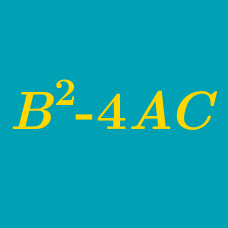Algebra

# Quadratic Discriminant: Level 3 Challenges

$$f(x)=ax^2+bx+c$$. $$a,b,c$$ are real numbers. Given that $$c(a+b+c)<0$$ , then what can we say about $$b^2-4ac$$ ?

Find the number of positive real roots of the equation $x^{4}-4x-1=0.$

Given a function $f(x) = ax^{2}-bx-16$ doesn't has 2 distinct real roots. Find the maximum value of $$4a-b$$.

$$\begin{equation} \displaystyle \prod_{k=1}^{999} (x^2-47x+k) = (x^2-47x+1)(x^2-47x+2)\dots(x^2-47x+999) \end{equation}$$

If the product of all real roots of the polynomial above can be expressed in the form $$n!$$, what is the value of $$n$$?

Find the number of real roots of the polynomial $$3x^{5} -25x^{3} +60x$$.

×

Problem Loading...

Note Loading...

Set Loading...